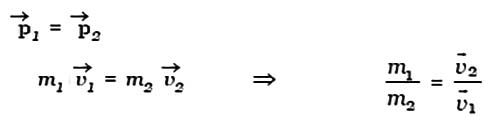# Describe Momentum of Body

Momentum of Body is observed experimentally that the force required to stop a moving object depends on two factors:

(i) mass of the body and

(ii) its velocity

A body in motion has momentum. The momentum of a body is defined as the product of its mass and velocity. If m is the mass of the body and ṽ, its velocity, the linear momentum of the body is given by Ƥ = mṽ.

Momentum has both magnitude and direction and it is, therefore, a vector quantity. The momentum is measured in terms of kg ms-1 and its dimensional formula is MLT-1.

When a force acts on a body. its velocity changes, consequently, its momentum also changes. The slowly moving bodies have smaller momentum than fast moving bodies of same mass.

If two bodies of unequal masses and velocities have same momentum, then,Hence for bodies of same momentum, their velocities are inversely proportional to their masses.